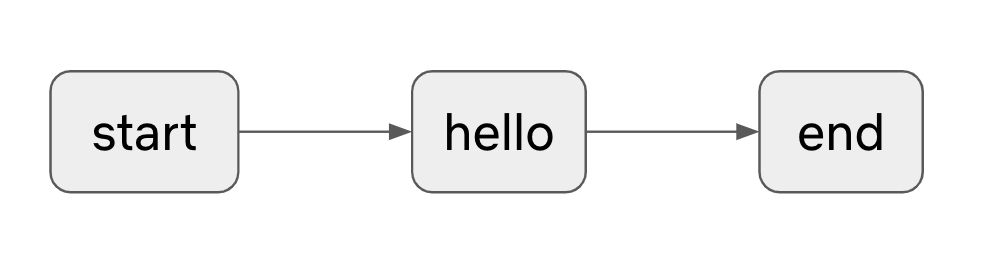# Episode 0: Hello World

## Metaflow says Hi!​

This flow is a simple linear workflow that verifies your installation by printing out Metaflow says: Hi!' to the terminal.

### To play this episode:​

If you haven't yet pulled the tutorials to your current working directory, you can follow the instructions here.

1. `cd tutorials/00-helloworld`
2. `Rscript helloworld.R show`
3. `Rscript helloworld.R run`The flow script below prints 'Hi' in Metaflow. Run this flow to validate that Metaflow is installed correctly.

helloworld.R
``library(metaflow)# This is the 'start' step. All flows must have a step named# 'start' that is the first step in the flow.start <- function(self){    print("HelloFlow is starting.")}# A step for metaflow to introduce itself.hello <- function(self){    print("Metaflow says: Hi!")}# This is the 'end' step. All flows must have an 'end' step,# which is the last step in the flow.end <- function(self){     print("HelloFlow is all done.")}metaflow("HelloFlow") %>%    step(step = "start",         r_function = start,         next_step = "hello") %>%    step(step = "hello",         r_function = hello,         next_step = "end") %>%    step(step = "end",         r_function = end) %>%    run()``